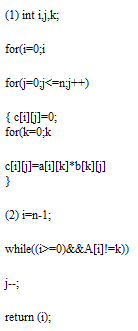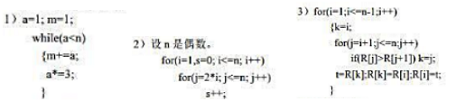# 求下列程序段的复杂度。###### 您可能感兴趣的试题
• 求下列程序段的复杂度。请帮忙给出正确答案和分析，谢谢！
• 求下列程序段的时间复杂度()。for(i=1;i<=n;i++)for(j=1;j<=n;j++)x=x+1;
A、O(n2)
B、O(n)
C、O(1)
D、O(0)

请帮忙给出正确答案和分析，谢谢！
• 写出下列各程序段关于n的时间复杂度。请帮忙给出正确答案和分析，谢谢！
• 堆排序的时间复杂度是O（）。

A、O（n）

B、O（2^n）

C、O（n^2）

D、O（nlogn）

• 链栈出栈的时间复杂度为（）

A、O(1)

B、O(n)

C、O(n^2)

D、以上都不对

• 执行下列程序的时间复杂度为O（mn），是否正确，回答对或错即可。 for(i=0;i<m;i++) for(j="0;j&lt;n;j++)" a[i][j]="i*j;&lt;br">
• 下列程序段的时间复杂度是()。s=i=0;do{i++;s+=i;}while(i<=n);
A、O(n)
B、O(log2(n))
C、O(n*log2(n))
D、O(n^2)

请帮忙给出正确答案和分析，谢谢！
• 以下程序段较为精确的时间复杂度为( )。 i=1; while(i<=n) i="i*3;&lt;br"> A、O(logn)

B、O(n)

C、O(n2)

D、O(n!)

• 下列程序段的时间复杂度为()。i=0，s=0;while(sn){s=s+i;i++;}

A、O(n)

B、O(n)

C、O(n)

D、O(n)

• n为正整数，下列程序段的时间复杂度是：______。 for（i=1, x=0; i<=n;> A、O(1)

B、O(n^2)

C、O(n)

D、O(2*n)亲，您的免费查看答案次数已用完了，请购买会员后再查看答案吧~微信支付支付宝支付### 请使用微信扫码支付(元)